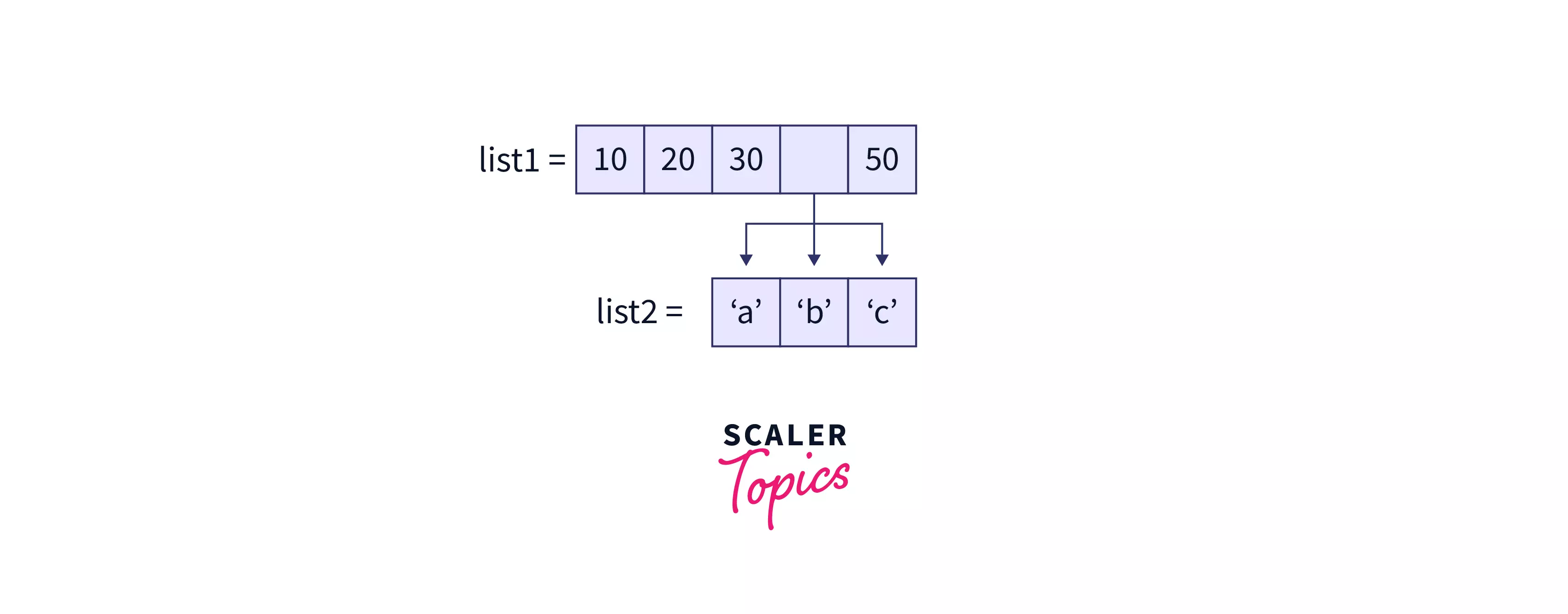# What is Nested List in Python?

Challenge Inside! : Find out where you stand! Try quiz, solve problems & win rewards!
Learn via video courses

Among all the data structures, list is the most common one and is widely used. But what if we need to insert or add a list inside the list? As we know, that list can contain any object, so here the concept of nested list is introduced.

So simply, when there is a list containing a list inside itself as an element(sublist) or data, then that list is known as a nested list. To get more clear with the nested list refer to an example given below.## How to Create a Nested List in Python?

Here is the code below for the example of how to create a nested list.

Code:

## Examples of Nested lists in Python

### Example 1: Access Nested List Items by Index

Multiple indexes can retrieve specific items in a nested list. An example for the same is given below.

Code:

Output:

### Example 2: Add Items to a Nested List

An item can be added to the nested list according to the conditions by using the methods given below.

By using the append() method to add additional values to the end of the nested list

Code:

Output:

By using the insert() method to insert an item at the given index in a nested list

Code:

Output:

By using the extend() method to combine two lists into one

Code:

Output:

### Example 3: Change Nested List Item Value

By referring to the index number of a specific item in the sublist, its value could be changed, as done below.

Code:

Output:

### Example 4: Remove Items from a Nested List

An item can be removed from the nested list according to the conditions by using the methods given below.

Using pop()

You can use the pop() method if you know the index of the element you want. This pop() method changes the list by removing that particular element from the list.

Code:

Output:

Using del

If there is no need of the element that is to be deleted, then del can be used, as done below.

Code:

Output

Using remove()

If an item's index is not known and is to be deleted, then remove() method can be used to delete by the value itself, as done below.

Code:

Output:

### Example 5: Iterate Through a Nested List

Use the simple for loop to iterate over the items in a nested list, as done below.

Code:

Output:

## Python Program to Flatten a Nested List

Now let's take a view on that how to create a flattened list from a nested list. The nested list can be flattened using the following methods.

### Example 6: Using List Comprehension

Code:

Output

This is one of the simplest ways to flatten a list in Python.

Here, list comprehension access the sublist from Nested_list, then access each element of the sublist. Then each item is stored in flattened_list.

### Example 7: Using Nested for Loops

Code:

Output:

Here, firstly create a new empty list named as flattened_list is created, then each item from the sublist is accessed using the nested for loop and append that item in the flattened list.

### Example 8: Using Itertools Package

Code:

Output:

Here, the python itertools module is used for flattening the nested list in which chain method returns each item of each iterable(sublist) from the nested list. After that list method is used to convert all those returned items into a list.

### Example 9: Using sum() method

Output

Here, the sum method takes 2 arguments those are Nested_list and an empty list in python i.e '[]'. Then after that sum method combines both Nested_list and empty list to create a new flattened list.

### Example 10: Using Lambda and reduce()

Code:

Output:

Here, the reduce() method is doing nothing but applying a lambda function over each iterable i.e., sublist of a nested list.

1. reduce() method- Reduce(fun,seq) method applies a specific function to all of the list items mentioned in the sequence passed along. The "functools" module contains the definition for this function.

2. lambda() method-Lambdafunctions take as many parameters as they like, but they can only return one value in terms of an expression.